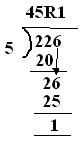Email us to get an instant 20% discount on highly effective K-12 Math & English kwizNET Programs!

Online Quiz (WorksheetABCD)

Questions Per Quiz = 2 4 6 8 10

Grade 3 - Mathematics10.10 Dividing Three Digit by Single Digit Number (WIZ Math)

 Method: Start dividing the dividend by the divisor. Look at the first number of the dividend. If this number is greater than divisor then divide it by the divisor. If this number is less than divisor then choose two digits and divide them by the divisor. Example:Look at the first digit of the dividend, it is 2. Since 2 is lesser than 4, include the next digit, that makes it 22. Now divide 22 by 4, the quotient is 5. Write 20 under 22, remainder is 2. Now bring down the third digit 6 and place it beside 2, that makes it 26. Divide 26 by 4, the quotient is 6 which gives 24. The remainder is 2. Hence the quotient is 56 and remainder is 2. Answer: 45R1 Directions: Divide the following. Also write at least 10 examples of your own.
 Question 1: This question is available to subscribers only! Question 2: This question is available to subscribers only! Question 3: This question is available to subscribers only! Question 4: This question is available to subscribers only!